• 2020-11-30 09:27:00

好好看看

一、排序函数

NumPy 提供了多种排序的方法。 这些排序函数实现不同的排序算法，每个排序算法的特征在于执行速度，最坏情况性能，所需的工作空间和算法的稳定性。 下表显示了三种排序算法的比较。

种类速度最坏情况工作空间稳定性

'quicksort'（快速排序）

1

O(n^2)

0

'mergesort'（归并排序）

2

O(n*log(n))

~n/2

'heapsort'（堆排序）

3

O(n*log(n))

0

numpy.sort()

numpy.sort() 函数返回输入数组的排序副本。函数格式如下：

numpy.sort(a, axis, kind, order)

参数说明：

a: 要排序的数组

axis: 沿着它排序数组的轴，如果没有数组会被展开，沿着最后的轴排序， axis=0 按列排序，axis=1 按行排序

kind: 默认为'quicksort'（快速排序）

order: 如果数组包含字段，则是要排序的字段

importnumpy as np

a= np.array([[3, 7], [9, 1]])print('我们的数组是：')print(a)print('\n')print('调用 sort() 函数：')print(np.sort(a))print('\n')print('按列排序：')print(np.sort(a, axis=0))print('\n')print('按行列排序：')print(np.sort(a, axis=1))print('\n')

输出结果：

我们的数组是：

[[3 7]

[9 1]]

调用 sort() 函数：

[[3 7]

[1 9]]

按列排序：

[[3 1]

[9 7]]

按行列排序：

[[3 7]

[1 9]]

在 sort 函数中，指定排序字段

importnumpy as np

dt= np.dtype([('name', 'S10'), ('age', int)])

a= np.array([("aaa", 21), ("ccc", 25), ("ddd", 17), ("bbb", 27)], dtype=dt)print('a数组：',a)print('按 name 排序：',np.sort(a, order='name'))

输出结果为：

a数组： [(b'aaa', 21) (b'ccc', 25) (b'ddd', 17) (b'bbb', 27)]

按 name 排序： [(b'aaa', 21) (b'bbb', 27) (b'ccc', 25) (b'ddd', 17)]

numpy.argsort()

numpy.argsort() 函数返回的是数组值从小到大的索引值。

importnumpy as np

x= np.array([3, 1, 2])print ('我们的数组是：')print(x)print ('\n')print ('对 x 调用 argsort() 函数：')

y=np.argsort(x)print(y)print ('\n')print ('以排序后的顺序重构原数组：')print(x[y])print ('\n')print ('使用循环重构原数组：')for i iny:print (x[i], end=" ")

输出结果为：

我们的数组是：对x 调用argsort()函数：以排序后的顺序重构原数组：使用循环重构原数组123

numpy.lexsort()

numpy.lexsort() 用于对多个序列进行排序。把它想象成对电子表格进行排序，每一列代表一个序列，排序时优先照顾靠后的列。

这里举一个应用场景：小升初考试，重点班录取学生按照总成绩录取。在总成绩相同时，数学成绩高的优先录取，在总成绩和数学成绩都相同时，按照英语成绩录取…… 这里，总成绩排在电子表格的最后一列，数学成绩在倒数第二列，英语成绩在倒数第三列。

importnumpy as np

nm= ('raju','anil','ravi','amar')

dv= ('f.y.', 's.y.', 's.y.', 'f.y.')

ind=np.lexsort((dv,nm))print ('调用 lexsort() 函数：')print(ind)print ('\n')print ('使用这个索引来获取排序后的数据：')print ([nm[i] + "," + dv[i] for i in ind])

输出结果为：

调用lexsort()函数：使用这个索引来获取排序后的数据：['amar, f.y.','anil, s.y.','raju, f.y.','ravi, s.y.']

上面传入 np.lexsort 的是一个tuple，排序时首先排 nm，顺序为：amar、anil、raju、ravi 。综上排序结果为 [3 1 0 2]。

importnumpy as np#录入了四位同学的成绩，按照总分排序，总分相同时语文高的优先

math = (10, 20, 50, 10)

chinese= (30, 50, 40, 60)

total= (40, 70, 90, 70)#将优先级高的项放在后面

ind =np.lexsort((math, chinese, total))for i inind:print(total[i],chinese[i],math[i])

输出，是按总成绩排序，相同时语文成绩优先级更高:

403010705020706010904050

msort、sort_complex、partition、argpartition

函数描述

msort(a)

数组按第一个轴排序，返回排序后的数组副本。np.msort(a) 相等于 np.sort(a, axis=0)。

sort_complex(a)

对复数按照先实部后虚部的顺序进行排序。

partition(a, kth[, axis, kind, order])

指定一个数，对数组进行分区

argpartition(a, kth[, axis, kind, order])

可以通过关键字 kind 指定算法沿着指定轴对数组进行分区

sort_complex 复数排序

importnumpy as npprint(np.sort_complex([5, 3, 6, 2, 1]))print(np.sort_complex([1 + 2j, 2 - 1j, 3 - 2j, 3 - 3j, 3 + 5j]))

输出结果：

[1.+0.j 2.+0.j 3.+0.j 5.+0.j 6.+0.j]

[1.+2.j 2.-1.j 3.-3.j 3.-2.j 3.+5.j]

partition 分区排序

importnumpy as np

a= np.array([3, 4, 2, 1])#将数组 a 中所有元素（包括重复元素）从小到大排列，3 表示的是排序数组索引为 3 的数字，比该数字小的排在该数字前面，比该数字大的排在该数字的后面

print(np.partition(a, 3))#小于 1 的在前面，大于 3 的在后面，1和3之间的在中间

print(np.partition(a, (1, 3)))

输出结果：

[2 1 3 4]

[1 2 3 4]

argpartition

找到数组的第 3 小（index=2）的值和第 2 大（index=-2）的值

importnumpy as np

arr= np.array([46, 57, 23, 39, 1, 10, 0, 120])print(arr[np.argpartition(arr, 2)])print(arr[np.argpartition(arr, -2)[-2]])

输出结果：

1057

同时找到第 3 和第 4 小的值。注意这里，用 [2,3] 同时将第 3 和第 4 小的排序好，然后可以分别通过下标  和  取得。

importnumpy as np

arr= np.array([46, 57, 23, 39, 1, 10, 0, 120])print(arr[np.argpartition(arr, [2,3])])print(arr[np.argpartition(arr, [2,3])])

输出结果：

10

23

二、条件筛选函数

numpy.argmax() 和 numpy.argmin()

numpy.argmax() 和 numpy.argmin()函数分别沿给定轴返回最大和最小元素的索引。

importnumpy as np

a= np.array([[30, 40, 70], [80, 20, 10], [50, 90, 60]])print('我们的数组是：')print(a)print('\n')

maxindex=np.argmax(a)print('调用 argmax() 函数：',maxindex)

minindex=np.argmin(a)print('调用 argmin() 函数：',minindex)print('展开数组：',a.flatten())print('展开数组中的最小值：',a.flatten()[minindex])print('展开数组中的最大值：',a.flatten()[maxindex])

maxindex= np.argmax(a, axis=0)print('沿轴 0 的最大值索引：',maxindex)

minindex= np.argmin(a, axis=0)print('沿轴 0 的最小值索引：',minindex)

maxindex= np.argmax(a, axis=1)print('沿轴 1 的最大值索引：',maxindex)

minindex= np.argmin(a, axis=1)print('沿轴 1 的最小值索引：',minindex)

输出结果为：

我们的数组是：

[[30 40 70]

[80 20 10]

[50 90 60]]

调用 argmax() 函数： 7

调用 argmin() 函数： 5

展开数组： [30 40 70 80 20 10 50 90 60]

展开数组中的最小值： 10

展开数组中的最大值： 90

沿轴 0 的最大值索引： [1 2 0]

沿轴 0 的最小值索引： [0 1 1]

沿轴 1 的最大值索引： [2 0 1]

沿轴 1 的最小值索引： [0 2 0]

numpy.nonzero()

numpy.nonzero() 函数返回输入数组中非零元素的索引。

importnumpy as np

a= np.array([[30,40,0],[0,20,10],[50,0,60]])print ('我们的数组是：')print(a)print ('\n')print ('调用 nonzero() 函数：')print (np.nonzero (a))

输出结果为：

我们的数组是：[]调用nonzero()函数：(array([0,0,1,1,2,2]),array([0,1,1,2,0,2]))

numpy.where()

numpy.where() 函数返回输入数组中满足给定条件的元素的索引。

importnumpy as np

x= np.arange(9.).reshape(3, 3)print('我们的数组是：')print(x)print('\n')

y= np.where(x > 3)print('大于 3 的元素的索引：')print(y)print('\n')print('使用这些索引来获取满足条件的元素：')print(x[y])

输出结果为：

我们的数组是：[[0.1.2.][3.4.5.][6.7.8.]]

大于3的元素的索引：(array([1,1,2,2,2]),array([1,2,0,1,2]))

使用这些索引来获取满足条件的元素：[4.5.6.7.8.]

numpy.extract()

numpy.extract() 函数根据某个条件从数组中抽取元素，返回满足条件的元素。

importnumpy as np

x= np.arange(9.).reshape(3, 3)print ('我们的数组是：')print(x)#定义条件, 选择偶数元素

condition = np.mod(x,2) ==0print ('按元素的条件值：')print(condition)print ('使用条件提取元素：')print (np.extract(condition, x))

输出结果为：

我们的数组是：[[0.1.2.][3.4.5.][6.7.8.]]

按元素的条件值：[[TrueFalseTrue][FalseTrueFalse][TrueFalseTrue]]

使用条件提取元素：[0.2.4.6.8.]

三、统计函数

NumPy 提供了很多统计函数，用于从数组中查找最小元素，最大元素，百分位标准差和方差等。

带轴向就是计算整行或整列的最大值和最小值，不带轴向，就是整个数组的最大值和最小值

numpy.amin() 和 numpy.amax()

numpy.amin() 用于计算数组中的元素沿指定轴的最小值。

numpy.amax() 用于计算数组中的元素沿指定轴的最大值。

importnumpy as np

a= np.array([[3, 7, 5], [8, 4, 3], [2, 4, 9]])print('我们的数组是：')print(a)print('\n')print('调用 amin() 函数：')print(np.amin(a, 1))print('\n')print('再次调用 amin() 函数：')print(np.amin(a, 0))print('\n')print('调用 amax() 函数：')print(np.amax(a, 1))print('\n')print('再次调用 amax() 函数：')print(np.amax(a, axis=0))print('\n')print('整个数组中最大和最小值为：')print(np.amin(a),np.amax(a))

输出结果为：

我们的数组是：

[[3 7 5]

[8 4 3]

[2 4 9]]

调用 amin() 函数：

[3 3 2]

再次调用 amin() 函数：

[2 4 3]

调用 amax() 函数：

[7 8 9]

再次调用 amax() 函数：

[8 7 9]

整个数组中最大和最小值为：

2 9

numpy.ptp()

numpy.ptp()函数计算数组中元素最大值与最小值的差（最大值 - 最小值）。

The name of the function comes from the acronym for 'peak to peak'

importnumpy as np

a= np.array([[3, 7, 5], [8, 4, 3], [2, 4, 9]])print('a数组：')print(a)print('\n')print('调用 ptp() 函数：',np.ptp(a))print('沿轴 1 调用 ptp() 函数：',np.ptp(a, axis=1))print('沿轴 0 调用 ptp() 函数：',np.ptp(a, axis=0))

输出结果为：

a数组：

[[3 7 5]

[8 4 3]

[2 4 9]]

调用 ptp() 函数： 7

沿轴 1 调用 ptp() 函数： [4 5 7]

沿轴 0 调用 ptp() 函数： [6 3 6]

numpy.percentile()

百分位数是统计中使用的度量，表示小于这个值的观察值的百分比。

numpy.percentile(a, q, axis)

参数说明：

a: 输入数组

q: 要计算的百分位数，在 0 ~ 100 之间

axis: 沿着它计算百分位数的轴

首先明确百分位数：

第 p 个百分位数是这样一个值，它使得至少有 p% 的数据项小于或等于这个值，且至少有 (100-p)% 的数据项大于或等于这个值。

举个例子：高等院校的入学考试成绩经常以百分位数的形式报告。比如，假设某个考生在入学考试中的语文部分的原始分数为 54 分。相对于参加同一考试的其他学生来说，他的成绩如何并不容易知道。但是如果原始分数54分恰好对应的是第70百分位数，我们就能知道大约70%的学生的考分比他低，而约30%的学生考分比他高。这里的 p = 70。

importnumpy as np

a= np.array([[10, 20, 30], [40, 50, 60], [70, 80, 90]])

p1= np.percentile(a, 50)

p2= np.percentile(a, 50, axis=1)

p3= np.percentile(a, 50, axis=0)print("a数组:\n",a,"\n")print("p1:", p1)print("p2:", p2)print("p3:", p3)

输出结果：

a数组:

[[10 20 30]

[40 50 60]

[70 80 90]]

p1: 50.0

p2: [20. 50. 80.]

p3: [40. 50. 60.]

importnumpy as np

a= np.array([[10, 7, 4], [3, 2, 1]])print('a数组是：')print(a)print ('\n')print('调用 percentile() 函数：',np.percentile(a, 50)) #50% 的分位数，就是 a 里排序之后的中位数

print('纵列调用 percentile() 函数：',np.percentile(a, 50, axis=0)) #axis 为 0，在纵列上求

print('横行调用 percentile() 函数：',np.percentile(a, 50, axis=1)) #axis 为 1，在横行上求

print ('\n')print('横行调用 percentile() 函数,保持维度不变：')print(np.percentile(a, 50, axis=1, keepdims=True)) #保持维度不变

输出结果为：

a数组是：

[[10 7 4]

[ 3 2 1]]

调用 percentile() 函数： 3.5

纵列调用 percentile() 函数： [6.5 4.5 2.5]

横行调用 percentile() 函数： [7. 2.]

横行调用 percentile() 函数,保持维度不变：

[[7.]

[2.]]

numpy.median()

numpy.median() 函数用于计算数组 a 中元素的中位数（中值）

importnumpy as np

a= np.array([[30,65,70],[80,95,10],[50,90,60]])print ('我们的数组是：')print(a)print ('\n')print ('调用 median() 函数：')print(np.median(a))print ('\n')print ('沿轴 0 调用 median() 函数：')print (np.median(a, axis =0))print ('\n')print ('沿轴 1 调用 median() 函数：')print (np.median(a, axis = 1))

输出结果为：

我们的数组是：[]调用median()函数：65.0沿轴0调用median()函数：[50.90.60.]沿轴1调用median()函数：[65.80.60.]

numpy.mean()

numpy.mean() 函数返回数组中元素的算术平均值。 如果提供了轴，则沿其计算。

算术平均值是沿轴的元素的总和除以元素的数量。

importnumpy as np

a= np.array([[1,2,3],[3,4,5],[4,5,6]])print ('我们的数组是：')print(a)print ('\n')print ('调用 mean() 函数：')print(np.mean(a))print ('\n')print ('沿轴 0 调用 mean() 函数：')print (np.mean(a, axis =0))print ('\n')print ('沿轴 1 调用 mean() 函数：')print (np.mean(a, axis = 1))

输出结果为：

我们的数组是：[]调用mean()函数：3.6666666666666665沿轴0调用mean()函数：[2.666666673.666666674.66666667]沿轴1调用mean()函数：[2.4.5.]

numpy.average()

numpy.average() 函数根据在另一个数组中给出的各自的权重计算数组中元素的加权平均值。

该函数可以接受一个轴参数。 如果没有指定轴，则数组会被展开。

加权平均值即将各数值乘以相应的权数，然后加总求和得到总体值，再除以总的单位数。

考虑数组[1,2,3,4]和相应的权重[4,3,2,1]，通过将相应元素的乘积相加，并将和除以权重的和，来计算加权平均值。

加权平均值 = (1*4+2*3+3*2+4*1)/(4+3+2+1)

importnumpy as np

a= np.array([1, 2, 3, 4])print('我们的数组是：')print(a)print('\n')print('不指定权重，调用 average() 函数：',np.average(a)) #不指定权重时相当于 mean 函数

wts= np.array([4, 3, 2, 1])print('指定权重后，调用 average() 函数：',np.average(a, weights=wts))#如果 returned 参数设为 true，同时返回权重的和

print('returned=True，调用 average() 函数：',)print(np.average([1, 2, 3, 4], weights=[4, 3, 2, 1], returned=True)) #权重和：4+3+2+1=10

输出结果为：

我们的数组是：

[1 2 3 4]

不指定权重，调用 average() 函数： 2.5

指定权重后，调用 average() 函数： 2.0

returned=True，调用 average() 函数：

(2.0, 10.0)

在多维数组中，可以指定用于计算的轴。

importnumpy as np

a= np.arange(6).reshape(3, 2)print('我们的数组是：')print(a)print('\n')

wt= np.array([3, 5])print('指定权重后，调用 average() 函数：')print(np.average(a, axis=1, weights=wt))print('\n')print('returned=True，调用 average() 函数：')print(np.average(a, axis=1, weights=wt, returned=True)) #返回每一行的权重

输出结果为：

我们的数组是：

[[0 1]

[2 3]

[4 5]]

指定权重后，调用 average() 函数：

[0.625 2.625 4.625]

returned=True，调用 average() 函数：

(array([0.625, 2.625, 4.625]), array([8., 8., 8.]))

标准差

标准差是一组数据平均值分散程度的一种度量。标准差是方差的算术平方根。

标准差=sqrt(((x1-x)^2 +(x2-x)^2 +......(xn-x)^2)/n)

#数组是 [1，2，3，4]，则其平均值为 2.5。 因此，差的平方是 [2.25,0.25,0.25,2.25]，并且差的平方加和后为5，再除以 4后开方，即 sqrt(5/4) ，结果为 1.1180339887498949。

importnumpy as npprint (np.std([1,2,3,4]))

输出结果为：

1.1180339887498949

方差

统计中的方差（样本方差）是每个样本值与全体样本值的平均数之差的平方值的平均数，即 mean((x - x.mean())** 2)。标准差是方差的平方根。

方差=((x1-x)^2 +(x2-x)^2 +......(xn-x)^2)/n

importnumpy as npprint (np.var([1,2,3,4]))

输出结果为：

1.25

更多相关内容
• 2007版EXCEL新增COUNTIFS函数的时候，我做了一次多条件重复计数的分析。公式不能简单地写成：SUM(1/COUNTIFS(A2:A14,A2:A14,B2:B14,B2:B14))具体可以参考《不重复计数迷思》由于COUNTIFS的性质，AB两列也不能先...2007版EXCEL新增COUNTIFS函数的时候，

我做了一次多条件不重复计数的分析。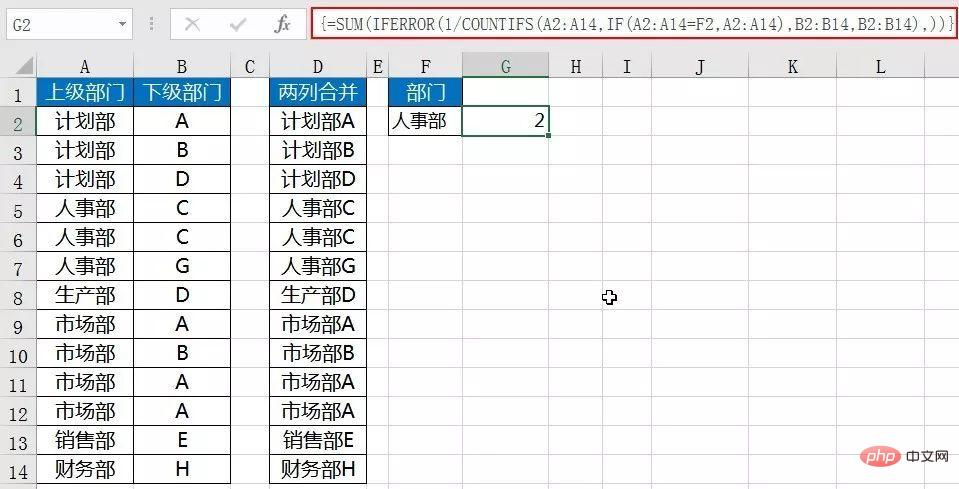公式不能简单地写成：

SUM(1/COUNTIFS(A2:A14,A2:A14,B2:B14,B2:B14))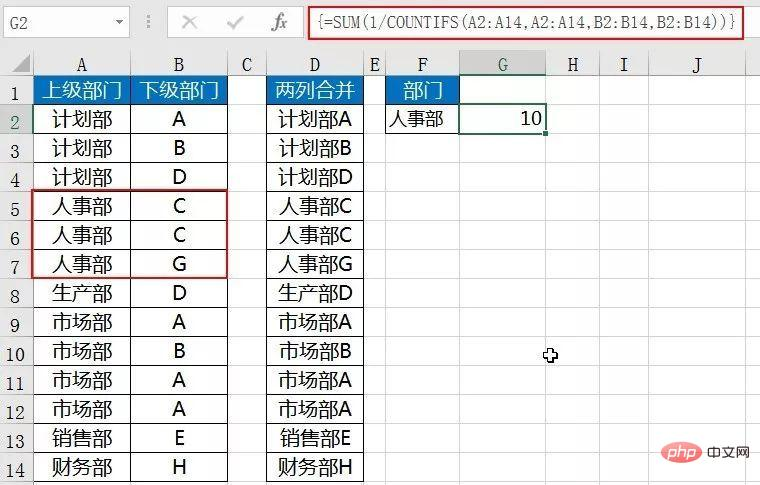具体可以参考《不重复计数迷思》

由于COUNTIFS的性质，AB两列也不能先连接起来再计算不重复数。

时隔十余年，微软又出了新函数UNIQUE。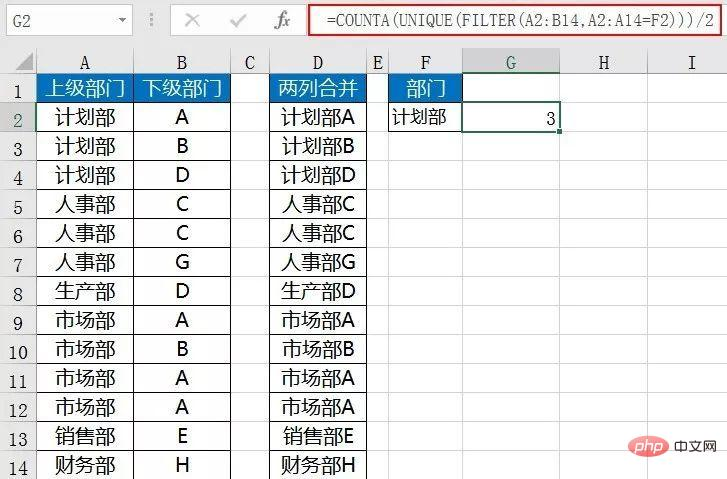公式利用新出筛选函数FILTER，按F2单元格条件筛选出数据。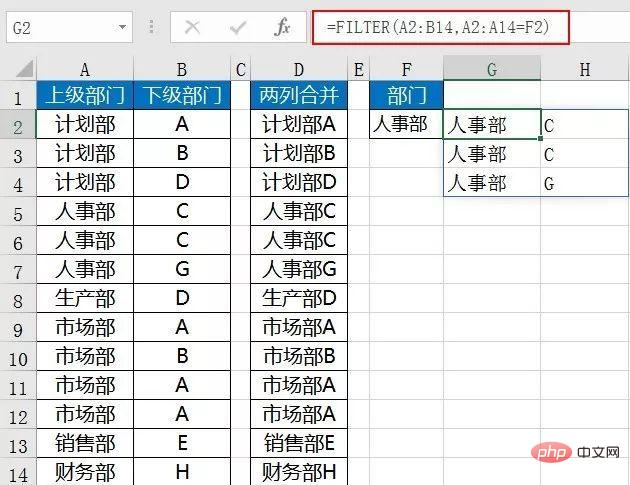再嵌套UNIQUE函数，提取出不重复数据。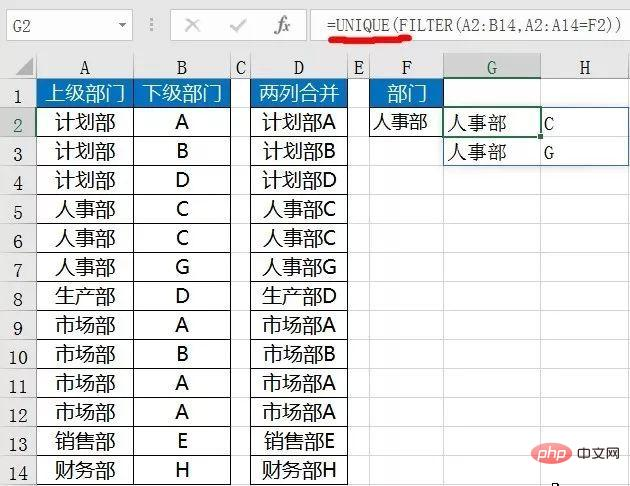最后用COUNT函数统计数据个数。

即使F2条件区域不是单值，

也可以利用FILTER函数筛选出符合条件的所有数据。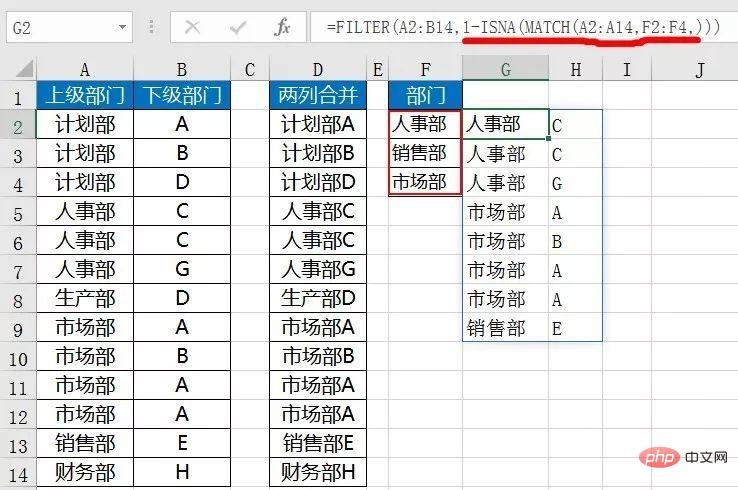本例公式MATCH部分也可以使用COUNTIF函数。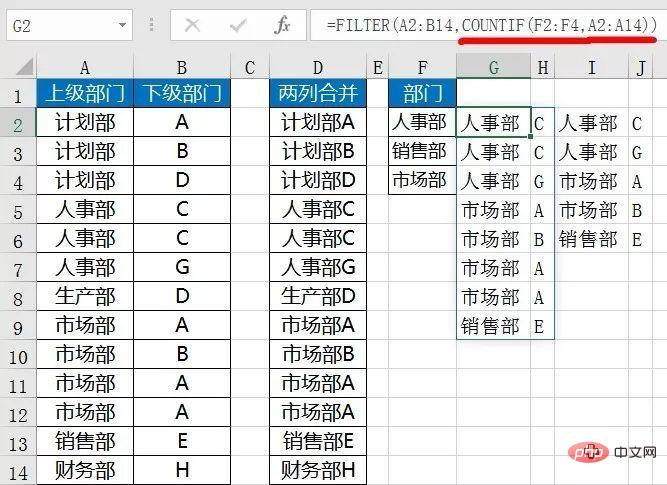然后嵌套UNIQUE函数提取不重复数据。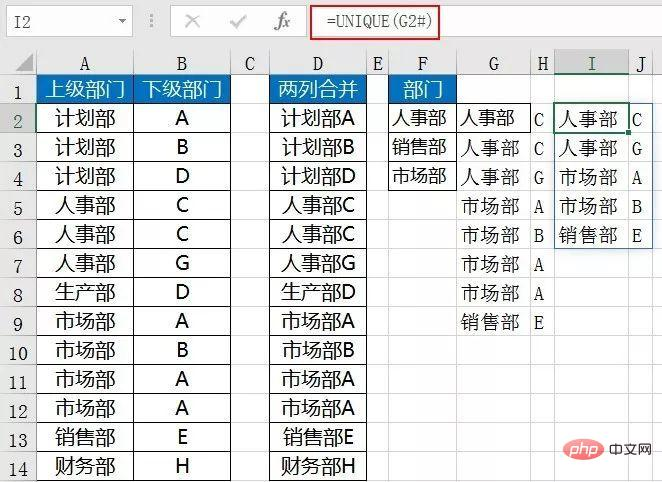微软原来的一些函数或多或少都有一些限制。

尤其类似COUNTIF这样的函数，第一个参数必须是区域引用。

现在新出的这几个函数，参数上都要友好的多。

所以这几个新函数的使用范围也就大许多。

最主要的新函数的增加降低了不少公式的难度。

更多Excel相关技术文章，请访问Excel教程栏目进行学习！

展开全文• 通过EXCEL VBA自定函数，简单快速地统计重复的个数，比如通过贷款明细账统计贷款户数等。
• 问题很常见，但是对新手来说又比较棘手，所以我们来一期，...需求：统计对应区域中不重复的人员个数？方法1：辅助列+函数这个方法推荐函数新手使用，帮助刚入门的同学解决问题！=SUMPRODUCT(($B$2:$B$15=$E2)/COUN... 问题很常见，但是对新手来说又比较棘手，所以我们来一期，系统讲讲，个人我个人的知识储备，大概写了8种，涵盖Excel的方方面面！最后一种也满足一下大家对于Python的好奇！ 友情提醒：应该是目前你所能见到的最全面的了！先收藏！ 需求：统计对应区域中不重复的人员个数？方法1：辅助列+函数 这个方法推荐函数新手使用，帮助刚入门的同学解决问题！=SUMPRODUCT(($B$2:$B$15=$E2)/COUNTIFS($A$2:$A$15,$A$2:$A$15))没有条件单纯的统计不重复内容，基本大家都知道使用SUM(1/COUNTIF(区域，区域))套路！如果有条件呢，我们可以把多个条件组合到一起，就变成一列了，然后使用单列不重复统计，最后再根据区域是的等于需要求的区域过滤！

如果你是一个函数高手，那么不用辅助列也可以！方法2：函数法(无辅助列)=SUMPRODUCT(($A$2:$A$15=$D2)/COUNTIFS($A$2:$A$15,$A$2:$A$15,$B$2:$B$15,$B$2:$B$15))这个应该不需要我过多的解释，只是把辅助列放到了公式中，单条件变多条件！ 好吧！你说函数法都太难了，有没有小白可以实现的，试试吧！方法3：透视表法(新手)要点说明：在创建透视表时 必须勾选添加到数据模型才可以使用不重复统计！ 其本质使用的是Power Pivot的不重复统计功能！ 如果觉得上面的方法过于小白，那我们可以试试Power Query方法4：Power Query点击 【转换】- 【数据分组】- 【非重复计数】 确定即可！在PQ中使用自带的分组功能，也能轻松搞定这样的需求！依旧不能再简单！ 如果你想稍微酷点，那么使用VBA怎么样？方法5：VBA 去重统计本是字典的强项，但是条件去重确实一点麻烦，不过可以使用双层字典即可轻松搞定！VBA爱好者，可以参考一下这种写法！方法6：Power Pivot-DAX度量值 在PP中使用DAX函数 DISTINCTCOUNT，可以非常简单的统计不重复的个数 写个度量值，扔到透视表值字段即可！其实到这里，也就差不多了，不要总是说去重统计不会！ 突然想起好久没写的SQL，好像我也懂一点点！，要不写一下SQL，顺便练个手！方法7：SQL法select 地区 , count(*) as 不重复人数 from (select distinct * from [data$] where 地区 <>null) group by 地区

先使用子查询去重，再进行分组聚合计数即可！有SQL大佬欢迎提供好的思路！好吧！最后我再写一个Python 就收工吧！方法8：番外-Pythondf.drop_duplicates().groupby('地区').count()

pandas在数据统计方面再次刷新了我的认知，一句搞定！不要鼓吹python

但是pandas确实是一个数据分析非常好用的库，谁用谁知道！本文由“壹伴编辑器”提供技术支持

8种方法，掏空了我自己，基本涵盖目前Excel的方方面面！也算是我个人的入门Excel后的一次小小的总结吧！

展开全文• 这次我们来介绍怎么在excel中实现多条件去重计数。 用到的函数有: 1.COUNTA() 即计算范围内的非空单元格数 2.UNIQUE() 计算范围内去重后的数组 3.FILTER 返回条件查询后的数组 如图，先用FILTER查询在A2:...

这次我们来介绍怎么在excel中实现多条件去重计数。

背景:我们需要计算出编号中为1，且时间中为2021年10月29日的配送中心有几个，抽象地来说我们需要对符合上述两个条件的配送中心进行计数。

用到的函数有:

1.COUNTA()

即计算范围内的非空单元格数

2.UNIQUE()

计算范围内去重后的数组

3.FILTER（）

返回多条件查询后匹配的数组。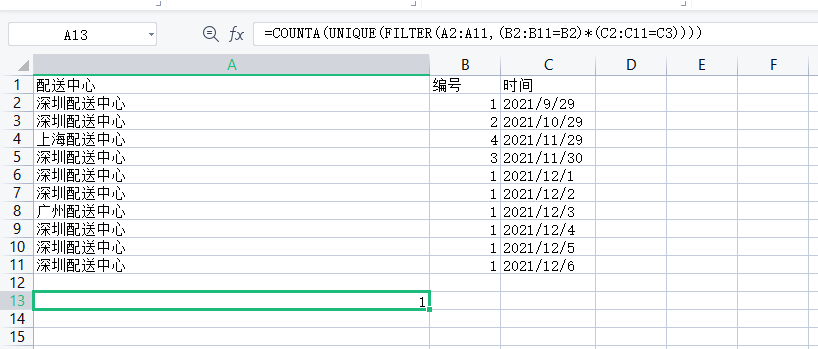如图，

1.先用FILTER查询在A2:A11中符合在B2:B11中等于B2的单元格，C2:C11中等于C3的单元格。（多对一查询）

2.接着用UNIQUE函数去重。（查询到的数组进行去重）

3.最后用COUNTA函数计算符合条件的单元格。（数组计数）

展开全文excel
•count distinct
• 利用Excel公式统计重复值的数量.rar,本例数据图表所示，要求统计A2:A11单元格区域中不重复记录的个数，有三种方法可以实现之。Excel应用
• 如果参数为数组，除了包含错误以外，可以使用任何其他类型的数据。 如果参数是单元格引用，还可以包含区域联合引用。 无论用户指定的参数是一维的数组还是二维的单元格引用，该函数都将返回一个垂直数组，即一...
• 在 Excel 中，CountIf函数用于统计指定范围内满足某个条件的单元格个数；...CountIf函数如果要实现多条件的的统计需要与 If、OffSet、Sum函数组合，并且有时条件需用数组，例如：Sum + If + CountIf函...
• Excel删除重复值统计重复次数、以及标记两列重复。excel
• 前段时间到一家制造型企业培训Excel，下课休息的时候，学员小Z向我咨询实际工作中的一个问题，以下是小Z的问题：表格有8000行数据（即8000个项目），其中一列的字段为合同编号，...曾经尝试用显示重复值功能，然后...
• Excel函数学习：悟空百问の009：去除重复项的深入思考我们经常整理一些数据，数据不可以动原数据，又需要进行数据分析...本期重点方案一：删除重复值操作流程：菜单栏-数据-删除重复值方法剖析：删除重复值有几个注...
• 转载自：玩转Excel条件格式，解决重复值一目了然，复杂工作变轻松 讲解之前，大家先复习一下countif这个函数，它用于对符合条件的单元格进行计数。=countif（区域，条件）。这个函数作为Excel基础函数之一，是日常...Excel
• 1、求和与统计函数 2、数字处理函数 3、日期与时间函数 4、查找与引用函数 5、逻辑与信息函数 6、文本处理函数 一、求和与统计函数 1、求和函数（sum） 案例1：求各品牌分别在每月和每个季度的销量合计 案例2：求...
• 1、我们知道，SQL语句中用count函数统计记录数量，配合distinct关键字可以统计非重复的记录数量。例如： select count(*), count(city_name), count(distinct city_name) from tb_county 查询结果是： 2534 2534 ...
• 本篇文章介绍一些常见的函数用法，sum（求和）、countrows（统计行数）、average（求平均）、lookupvalue（匹配）、related对一匹配、relatedtable一对匹配等，简化数据分析步骤提升效率 文章中的数据来
• MySql统计函数COUNT详解1. COUNT()函数概述2. COUNT()参数说明3. COUNT()使用场景4. COUNT()阿里开发规范 1. COUNT()函数概述 COUNT() 是一个聚合函数，返回指定匹配条件的行数。开发中常用来统计表中数据，全部数据...
• 广告关闭腾讯云11.11云上盛惠 ，精选热门产品助力上云，云服务器首年88元起，买的越返的越，最高返5000元！一般用小写字母和单下划线、数字等组合，有人习惯用aabb的样式，但我不推荐def是定义函数的关键词，这...
• 当数据量大的时候，快速把重复值找出来，是很多人非常头疼的问题。 我用的版本是 Excel 2016，其他版本的界面可能略有不同。 需求： 单独一列数据如何快速查重？ 列数据组合条件快速查重，如何实现？ ...excel
•         ...其中，前者用于标记是否有重复值，后者用于删除重复值，它们的判断标准是一样的，即只要两条数据中所有条目的值完全相等，就判断为重复值。 ...python
• 读数据import pandas as pddata = pd.read_csv('D:\git\python\code\第5章\产品统计表.csv')print(data)输出编号 产品 成本价(元/个) 销售价(元/个) 数量(个) 成本(元) 收入(元) 利润(元)0 a001 背包 16 65 60 960....
• 当同一个电话，被同一个回访人次回访时，只选其中一条用于回访统计。 选择的标准为：按照（序号）的优先级，3>2>1。同级别时，则选择其中任意一条。 重复记录标记为：1，不是重复记录标记为：0 实现效果如下： ...
• 要求从货运明细中统计各省某日发货点数量，公式如下： =SUMPRODUCT((Sheet1!$L$2:$L$1747=B6) *(Sheet1!$M$2:$M1747=$A$1)/ COUNTIF(Sheet1!$U$2:$U$1747,Sheet1!$U$2:$U\$1747)) Sheet1是货运明细，其中L列是发货...
• SUM函数返回表达式中所有值的和或仅非重复值的和。SUM 只能用于数字列。空值将被忽略。 参数说明： ALL：对所有的值应用此聚合函数。ALL是默认值。 DISTINCT：指定 SUM 返回唯一值的和。SUM (DISTINCT(字段名)) ..sql 数据库
• 今天来介绍EXCEL中的计算统计函数。我们大致分成7类来介绍统计函数。1. 求和SUM,SUMIF,SUMIFSSUM(Number1,[Number2],……)：计算单元格区域中所有数值的和SUMIF(range,criteria,[sum_range])；对满足的单元格...
• 巧用Hive自带函数进行字段分别去重计数统计 1-group by 和 distinct 大前提：大表统计能使用group by就不要使用distinct！！ 尤其是在数据量非常大的时候，distinct会将所有的统计信息加载到一个reducer里...Hive distinct
• EXCEL中的计算统计函数。我们大致分成7类来介绍统计函数。 1. ** 求和SUM,SUMIF,SUMIFS** SUM(Number1,[Number2],……)：计算单元格区域中所有数值的和 SUMIF(range,criteria,[sum_range])；对满足的单元格求和 ...
• 在使用mysql时，有时需要查询出某个字段不重复的记录，虽然mysql提供有distinct这个关键字来过滤掉多余的重复记录只保留一条，但往往只用它来返回不重复记录的条数，而不是用它来返回不重记录的所有。其原因是...
• 聚合函数又称为统计函数或汇总函数，它对一组进行计算并返回一个数值，是 SQL 中国能使用较的一类函数，对数据实现更加丰富的操作； 聚合函数常与 SELECT 语句一起使用； 2. 聚合函数 聚合函数对一组进行计算...sqlserver 数据库...